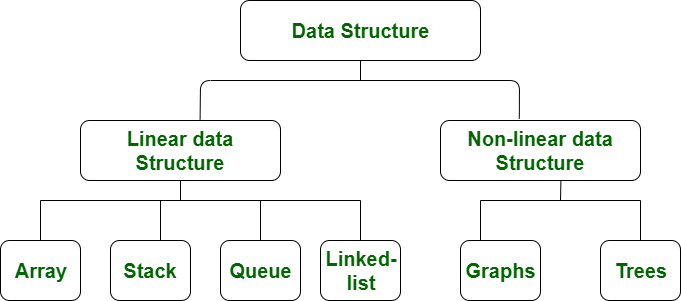# Difference between Linear and Non-linear Data Structures

Linear Data Structure:
Data structure where data elements are arranged sequentially or linearly where each and every element is attached to its previous and next adjacent is called a linear data structure. In linear data structure, single level is involved. Therefore, we can traverse all the elements in single run only. Linear data structures are easy to implement because computer memory is arranged in a linear way. Its examples are array, stack, queue, linked list, etc.

1. Array

The array is a type of data structure that stores elements of the same type. These are the most basic and fundamental data structures. Data stored in each position of an array is given a positive value called the index of the element. The index helps in identifying the location of the elements in an array.

If supposedly we have to store some data i.e. the price of ten cars, then we can create a structure of an array and store all the integers together. This doesn’t need creating ten separate integer variables. Therefore, the lines in a code are reduced and memory is saved. The index value starts with 0 for the first element in the case of an array.

2. Stack

The data structure follows the rule of LIFO (Last In-First Out) where the data last added element is removed first. Push operation is used for adding an element of data on a stack and the pop operation is used for deleting the data from the stack. This can be explained by the example of books stacked together. In order to access the last book, all the books placed on top of the last book have to be safely removed.

3. Queue

This structure is almost similar to the stack as the data is stored sequentially. The difference is that the queue data structure follows FIFO which is the rule of First In-First Out where the first added element is to exit the queue first. Front and rear are the two terms to be used in a queue.

Enqueue is the insertion operation and dequeue is the deletion operation. The former is performed at the end of the queue and the latter is performed at the start end. The data structure might be explained with the example of people queuing up to ride a bus. The first person in the line will get the chance to exit the queue while the last person will be the last to exit.

Linked lists are the types where the data is stored in the form of nodes which consist of an element of data and a pointer. The use of the pointer is that it points or directs to the node which is next to the element in the sequence. The data stored in a linked list might be of any form, strings, numbers, or characters. Both sorted and unsorted data can be stored in a linked list along with unique or duplicate elements.

5. Hash Tables

These types can be implemented as linear or non-linear data structures. The data structures consist of key-value pairs.

Non-linear Data Structure:
Data structures where data elements are not arranged sequentially or linearly are called non-linear data structures. In a non-linear data structure, single level is not involved. Therefore, we can’t traverse all the elements in single run only. Non-linear data structures are not easy to implement in comparison to linear data structure. It utilizes computer memory efficiently in comparison to a linear data structure. Its examples are trees and graphs.

1. Trees

A tree data structure consists of various nodes linked together. The structure of a tree is hierarchical that forms a relationship like that of the parent and a child. The structure of the tree is formed in a way that there is one connection for every parent-child node relationship. Only one path should exist between the root to a node in the tree. Various types of trees are present based on their structures like AVL tree, binary tree, binary search tree, etc.

2. Graph

Graphs are those types of non-linear data structures which consist of a definite quantity of vertices and edges. The vertices or the nodes are involved in storing data and the edges show the vertices relationship. The difference between a graph to a tree is that in a graph there are no specific rules for the connection of nodes. Real-life problems like social networks, telephone networks, etc. can be represented through the graphs.Difference between Linear and Non-linear Data Structures:

Feeling lost in the world of random DSA topics, wasting time without progress? It's time for a change! Join our DSA course, where we'll guide you on an exciting journey to master DSA efficiently and on schedule.
Ready to dive in? Explore our Free Demo Content and join our DSA course, trusted by over 100,000 geeks!